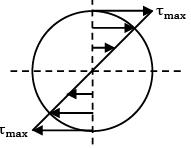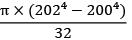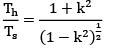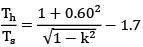Courses

# Test: Torsion Level - 1

## 10 Questions MCQ Test Strength of Materials (SOM) | Test: Torsion Level - 1

Description
This mock test of Test: Torsion Level - 1 for Mechanical Engineering helps you for every Mechanical Engineering entrance exam. This contains 10 Multiple Choice Questions for Mechanical Engineering Test: Torsion Level - 1 (mcq) to study with solutions a complete question bank. The solved questions answers in this Test: Torsion Level - 1 quiz give you a good mix of easy questions and tough questions. Mechanical Engineering students definitely take this Test: Torsion Level - 1 exercise for a better result in the exam. You can find other Test: Torsion Level - 1 extra questions, long questions & short questions for Mechanical Engineering on EduRev as well by searching above.
QUESTION: 1

Solution:
QUESTION: 2

### Polar section modulus is the ratio of

Solution: Polar section modulus, =Polar moment of inertia of the shaft / Maximum radius

QUESTION: 3

### Torsional stiffness is defined as

Solution:

Torsional rigidity of a shaft is defined as the torque required to produce a twist of one radian per unit length of the shaft.

QUESTION: 4

A shaft of circular section is said to be in pure torsion when it is subjected to equal and opposite end couples whose axes coincides with the

Solution: Torsion is about the polar centroidal axis i.e., along the axis of shaft

QUESTION: 5

In a solid circular shaft subjected to pure torsion, the maximum shear stress occurs at

Solution:
QUESTION: 6

Shear stress for a circular shaft due to torque varies

Solution:QUESTION: 7

The torque that produces a twist of one radian in a shaft per unit length is called

Solution: Torsional rigidity = CJ Units: kg cm2(or) N­mm2

QUESTION: 8

The torsional rigidity of a tube of thickness 1.0 mm, diameter 200 mm and rigidity modulus 100.0 GPa is

Solution: Torsional rigidity,

GJ = 100 × 103 ×= 637.8 × 109N­m2

= 637.8GN­m2

QUESTION: 9

A hollow shaft of same cross-section area and same mass as a solid shaft, transmits

Solution: If area of solid and hollow section are equalWhere

k = d / D

Eg: k=0.60Th = 1.7 Ts Hollow shaft have 70% more torque.

QUESTION: 10

If the moment of inertia of a section about its orthogonal axes are 1 × 106 and 2 × 106 and its area is 1000 units. Its polar moment of inertia is

Solution: Polar moment of inertia

Izz = Ixx + Iyy

= 1 × 106 + 2 × 106

Izz = 3 × 106